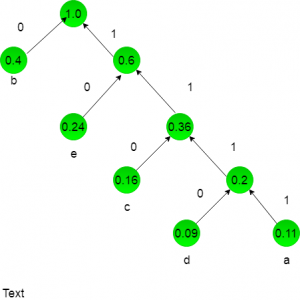Related Articles
UGC-NET | UGC-NET CS 2017 Nov – III | Question 35
• Last Updated : 20 Mar, 2018

A text is made up of the characters a, b, c, d, e each occurring with the probability 0.11, 0.40, 0.16, 0.09 and 0.24 respectively. The optimal Huffman coding technique will have the average length of:
(A) 2.40
(B) 2.16
(C) 2.26
(D) 2.15

Explanation: a = 0.11
b = 0.40
c = 0.16
d = 0.09
e = 0.24
we will draw a huffman tree:now huffman coding for character:

``` a = 1111
b = 0
c = 110
d = 1111
e = 10
lenghth for each character = no of bits * frequency of occurence:
a = 4 * 0.11
= 0.44
b = 1 * 0.4
=  0.4
c = 3 * 0.16
= 0.48
d = 4 * 0.09
=  0.36
e = 2 * 0.24
= 0.48
Now add these lenght for average length:
0.44 + 0.4 + 0.48 + 0.36 + 0.48 = 2.16
```

So, option (B) is correct.

My Personal Notes arrow_drop_up
Recommended Articles
Page :